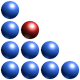# What are the Units of Complexity?Complexity is a fundamental physical characteristic of every system that may be found in Nature. Its importance is similar to that of energy. There have been numerous attempts of measuring complexity. However, most are limited either to very simple systems, character strings or to computer code and have very little practical use. In many cases, measures of complexity have been equated to entropy. Other approaches attempt to measure the degree of ’complicatedness’ of a system by analyzing the topology (structure) of graphs which connect system components. The first comprehensive measure of complexity for generic systems has been developed in 2003 by the J. Marczyk in the context of the Quantitative Complexity Theory, QCT, and introduced in 2009. The measure, which, unlike conventional attempts to quantify complexity, is bounded, is the first one which takes into account both entropy and structure as well as interactions between system components. Metrics which, for example, are based on part or component count, are unbounded and are therefore physically irrelevant.

The functionality of a given system is proportional to its complexity. More complex systems are able to perform more functions, a characteristic which is evident in our evolving biosphere. However, high complexity implies also an increase of management effort and energy necessary to govern a given system. When taken to extremes, excessive complexity becomes a formidable source of vulnerability. This is because excessively complex systems are capable of delivering surprising behaviour due to the fact that they can potentially function in a large number of modes.

Complexity is defined formally as a scalar function of structure and entropy:

C=f(S; E)

where S represents the so-called adjacency matrix and E is the entropy matrix. Entropy is computed based on Shannon´s formulation and expresses in bits the average amount of information. The above complexity formula measures the total amount of structured information in a system described by N variables and its unit is bit, which we call ´complexity bit´ or simply ´cbit´.

In the example below, relative to a corporation,we may say the the complexity of the business is 11.08 cbits, its critical complexity is 12.38 cbits and the lower complexity bound is 3.78 cbits.

www.ontonix.com